### Home > CALC > Chapter 4 > Lesson 4.4.2 > Problem4-139

4-139.
1. For f(x) = sin(x 2),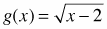, and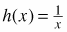, find the following functions and their domains. Homework Help ✎

1. f(g(x))

2. f(g(h(x)))

3. h(f(g(x)))

4. h(h(x))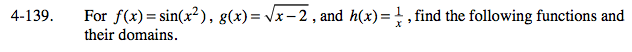$\text{sin}\left ( (\sqrt{x-2})^{2} \right )=$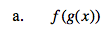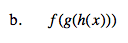Careful: f(g(h(x))) has a restricted domain.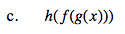$h(\text{part (a)})=\frac{1}{\text{(part (a))}}=$

Domain: Denominator cannot equal zero. For what value(s) of x does sin(x − 2) = 0? Also, due to the square root in the original function, x – 2 ≥ 0.

Domain: x > 2 and x ≠ 2+,πn, where n is an integer value.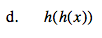$h(h(x))=\frac{1}{\frac{1}{x}}$

Simplify!